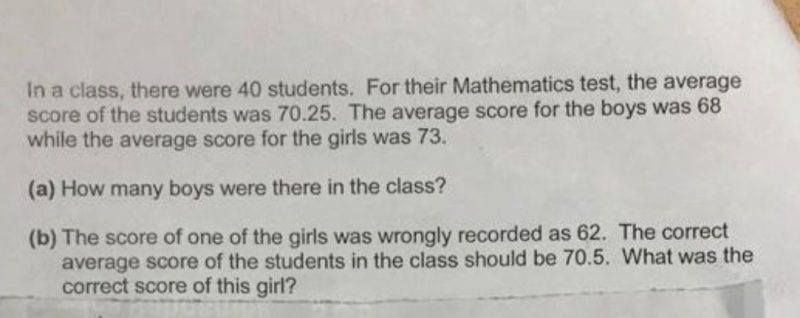Question**Dear Teachers, please help to solve the question. Thank you!

Total marks for all the pupils in the class— 40 x 70.25

=2810

Number of boys in     Number of girls   Total score of boys    Total score of girls     total score

the class.                             in the class

1.                                       39.                              1 x 68 =68.             39×73=2847       2847+68=2915
2.                                         38.                             2 x 68=136.            38×73=2774.    2774+136=2910
3.                                           37.                             3×68=204.              37×73=2701  2701+204=2905

Difference is 2915-2910 = 5

2915-2810=105

105/5=21

1+21=22

(a) 22

70.5×40=2820

2820-2810=10

10+62=72

(b)72

0 Replies 1 Like ✔Accepted Answer